summary of site-wide JavaScript functionalityUnited States-English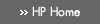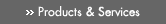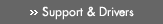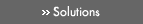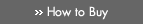» Contact HPSearch:More optionsManualTechnical documentation - EnglishAll of HP USHP-UX Reference > R# rint(3M)

HP-UX 11i Version 3: February 2007»

## Technical documentation» Feedback## » Index## NAME

rint(), rintf(), rintl(), rintw(), rintq(), nearbyint(), nearbyintf(), nearbyintl(), nearbyintw(), nearbyintq() — round to nearest integer functions

## SYNOPSIS

#include <math.h>

double rint(double x);

double nearbyint(double x);

### HP Integrity Server Only

float rintf(float x);

long double rintl(long double x);

extended rintw(extended x);

float nearbyintf(float x);

long double nearbyintl(long double x);

extended nearbyintw(extended x);

## DESCRIPTION

rint() and nearbyint() return the integer (represented as a floating-point number) nearest x according to the current rounding direction mode. These functions meet the requirement of the IEEE-754 standard for an operation that rounds to integer in floating-point format.

The two functions are identical except that rint() raises the inexact exception if the result differs in value from the argument, while nearbyint() does not.

In the default rounding direction (round to nearest), rint(x) is the integer nearest x with the additional stipulation that if |rint(x) -x|=1/2, then rint(x) is even. (The same statement applies to nearbyint(x).)

If the current rounding direction is toward negative infinity, rint() and nearbyint() return the same value as floor(). If the current rounding direction is toward positive infinity, rint() and nearbyint() return the same value as ceil().

### Integrity Server Only

rintf()and nearbyintf() are float versions of rint() and nearbyint() respectively; they take a float argument and return a float result.

rintl() and nearbyintl() are long double versions of rint() and nearbyint() respectively; they take a long double argument and return a long double result.

rintw() and nearbyintw() are extended versions of rint() and nearbyint() respectively; they take an extended argument and return an extended result.

rintq() and nearbyintq() are equivalent to rintl() and nearbyintl() respectively on HP-UX systems.

## USAGE

To use these functions, compile either with the default -Ae option or with the -Aa and -D_HPUX_SOURCE options. To use (for Integrity servers) ceilw(), nearbyintw(), ceilq(), or nearbyintq(), compile with the -fpwidetypes option. Make sure your program includes <math.h>. Link in the math library by specifying -lm on the compiler or linker command line.

For more information, see the HP-UX floating-point guide for HP Integrity servers at the following site: http://www.hp.com/go/fp.

## RETURN VALUE

If x is ±INFINITY or ±zero, rint() and nearbyint() return x.

If x is NaN, the rint() and nearbyint() functions return NaN.

## ERRORS

No errors are defined.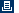Printable versionPrivacy statement Using this site means you accept its terms Feedback to webmaster© 1983-2007 Hewlett-Packard Development Company, L.P.